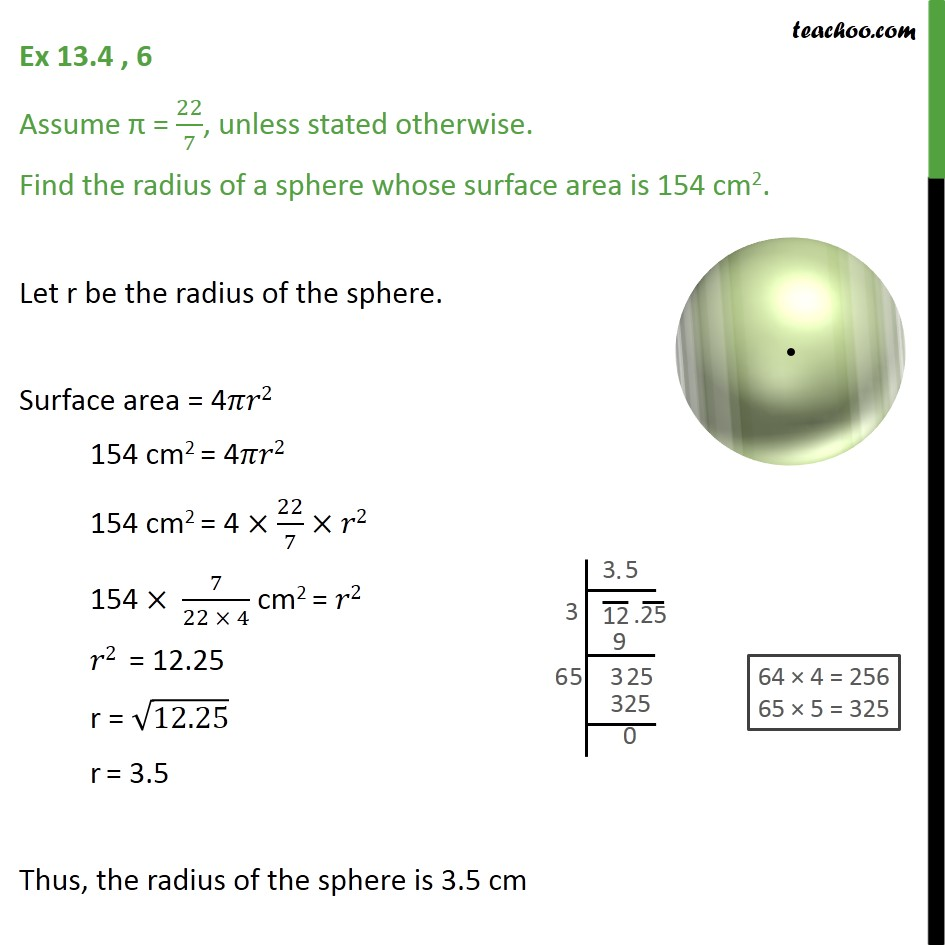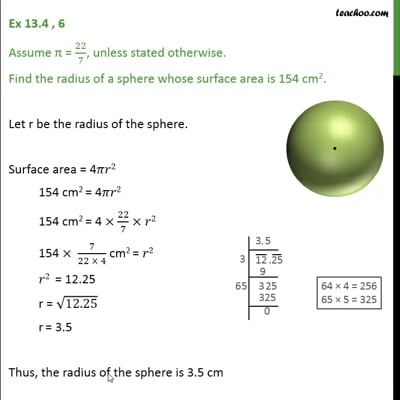Ex 13.4

Chapter 13 Class 9 Surface Areas and Volumes
Serial order wiseThis video is only available for Teachoo black users

Solve all your doubts with Teachoo Black (new monthly pack available now!)

### Transcript

Ex 13.4 , 6 Assume = 22/7, unless stated otherwise. Find the radius of a sphere whose surface area is 154 cm2. Let r be the radius of the sphere. Surface area = 4 2 154 cm2 = 4 2 154 cm2 = 4 22/7 2 154 7/(22 4) cm2 = 2 2 = 12.25 r = 12.25 r = 3.5 Thus, the radius of the sphere is 3.5 cm